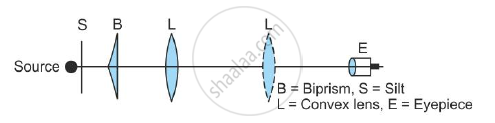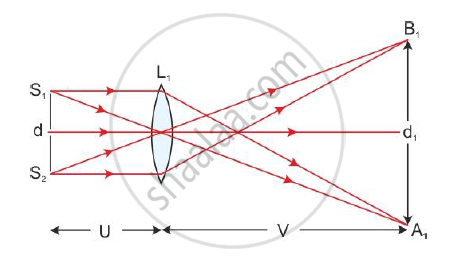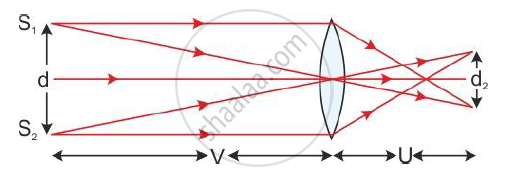HSC Science (Computer Science) 12th Board ExamMaharashtra State Board
Share

Describe Biprism Experiment to Calculate the Wavelength of a Monochormatic Light. Draw the Necessary Ray Diagram. - HSC Science (Computer Science) 12th Board Exam - Physics

ConceptMeasurement of Wavelength by Biprism Experiment

Question

Describe biprism experiment to calculate the wavelength of a monochormatic light. Draw the necessary ray diagram.

Solution

To measure the wavelength of light, an optical bench is used. It is about one and a half metre long, and a scale is marked along its length. Four adjustable stands carrying the slit (S), biprism (B), lens (L) and micrometre eyepiece (E) are mounted on the optical bench.Initially, the slit, biprism and eyepiece are kept at the same height such that their centres are in the same line. The slit is made narrow and is illuminated by a sodium vapour lamp. The biprism is now rotated slowly about a horizontal axis so that its refracting edge becomes parallel to the slit.

When the refracting edge of the biprism becomes exactly parallel, the interference pattern consisting of alternate bright and dark bands appear in the field of view of the eyepiece.

The formula to be used is

lambda= (Xd)/D

To determine the wavelength, the following steps are taken:

(1) The distance between the slit and the eyepiece D can be easily measured from the scale marked on the optical bench.

(2) The bandwidth X is measured with the help of the micrometre eyepiece. The vertical crosswire in the eyepiece is adjusted at the centre of the bright fringe. The micrometre eyepiece reading is noted.

Now, the eyepiece is moved horizontally until the crosswire has moved over a known number N of bright fringes. Again the reading of the micrometre eyepiece is noted. The difference between the two readings of the micrometre eyepiece gives the distance x through which the eyepiece is moved. Thus, the average distance between two adjacent bright fringes is

X=x/N

(3) The distance ‘d’ between two coherent sources cannot be measured directly because the sources are virtual. Hence, the method of conjugate foci is used. In this method, the object and image distances get interchanged in two adjustments.The convex lens of short focal length is introduced between the biprism and the eyepiece. Without disturbing the slit and biprism, the eyepiece is moved back so that its distance from the slit becomes greater than four times the focal length of the lens.

The lens is moved towards the slit and its position L1 is so adjusted that two magnified images A1 and B1 of S1 and S2 are formed in the focal plane of the eyepiece. The distance d1 between A1 and B1 is measured by the micrometre eyepiece.

From the figure, we get

"Size of image"/"Size of object" =" Distance of image"/"Distance of object"

d_1/d=v/u ------(1)The lens is now moved towards the eyepiece to the position L2 where two diminished images A2 and B2 of S1 and S2 are formed in the focal plane of the eyepiece.

The distance d2 between A2 and B2 is measured by the micrometre eyepiece. Then by the principle of conjugate foci, we can write

d_2/d=u/v -------------(2)

Multiplying equations (1) and (2), we get

(d_1d_2)/d_2=v/uxxu/v=1

therefore d^2=d_1d_2

therefore d=sqrt(d_1d_2)

Thus, knowing D, X and d, we can calculate the wavelength λ of monochromatic light by using the equation λ=Xd/D

The critical angle is given as

sin theta_c=1/n

It is given that

theta_c=sin^-1(3/5)

therefore 1/n=3/5

therefore n=5/3

Now, the polarising angle is given as

theta_p=tan^-1n=tan^-1(5/3)

Is there an error in this question or solution?

APPEARS IN

2014-2015 (March) (with solutions)
Question 8.2 | 7.00 marks
2016-2017 (March) (with solutions)
Question 8.1 | 7.00 marks
Solution Describe Biprism Experiment to Calculate the Wavelength of a Monochormatic Light. Draw the Necessary Ray Diagram. Concept: Measurement of Wavelength by Biprism Experiment.
S